# Walk me through a DCF

A guaranteed question in your investment banking interviewsReviewed by

Sid Arora

Expertise: Investment Banking | Hedge Fund | Private Equity

Updated:

October 6, 2023

"Walk me through a DCF" is a question you can be sure to face if you are recruiting for investment banking or any other front office role. This guide will break the question into key segments, with brief explanations of the relevant math formulas if you have time to get into them.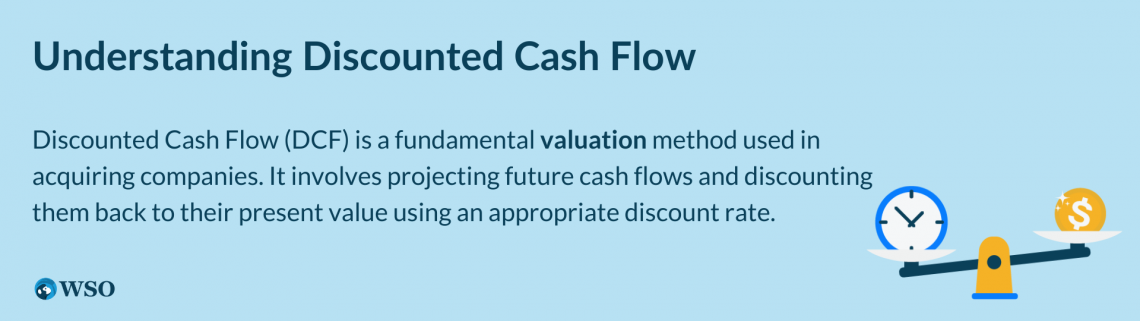DCFs are critical to a firm’s valuation process in acquiring a company. A basic DCF model’s function is to project future cash flows and then discount them back to their present values at a realistic discount rate that reflects the riskiness of the capital.

The most crucial step of a DCF is dividing equity value by diluted outstanding shares to derive the equity value per share of the company. The equity value per share is then compared to the market value to see if a company is under or overvalued.

You should note that DCFs work better in education than in practice. In education, the syllabus can create cookie-cutter economic situations where your assumptions do not fail. In reality, it is tough to make flawless assumptions to evaluate equities.

This is because DCF values rely upon future estimations of cash flow, which can cause inaccuracies if unexpected events occur. Therefore, it is essential to note that a DCF is not the only important investment analysis method.

Remember, a good investment analysis relies on many methods to reach a strong and consistent conclusion. Let's start by understanding what a DCF Model is before moving on to the section on answering the interview question.

## What is a DCF?

There is no better way than using DCFs to calculate a company’s intrinsic value. Renowned investors such as Ackman and Buffett have contended that the expected future net cash flow is fundamental to determining a company’s value.

“Let’s start with intrinsic value, an all-important concept that offers the only logical approach to evaluating the relative attractiveness of investments and businesses. Intrinsic value can be defined simply as the discounted value of the cash that can be taken out of a business during its remaining life.”

Ackman, in an interview:### The Only Program You Need to Land in High Finance Careers

The most comprehensive curriculum and support network to break into high finance.

“If we can’t predict the cash flows, we don’t know what it’s worth. And if there’s a wide gap between price and value, we can buy it for 50 cents when it’s worth \$1.20 - then we take a good look at why that is.”

The DCF model is helpful for someone planning to invest as they gain insight into the profitability of their investment. For example, if the intrinsic value calculated in a DCF is higher than the value of an investment, it could represent positive returns and optimal investment.

## How to Answer "Walk me through a DCF?"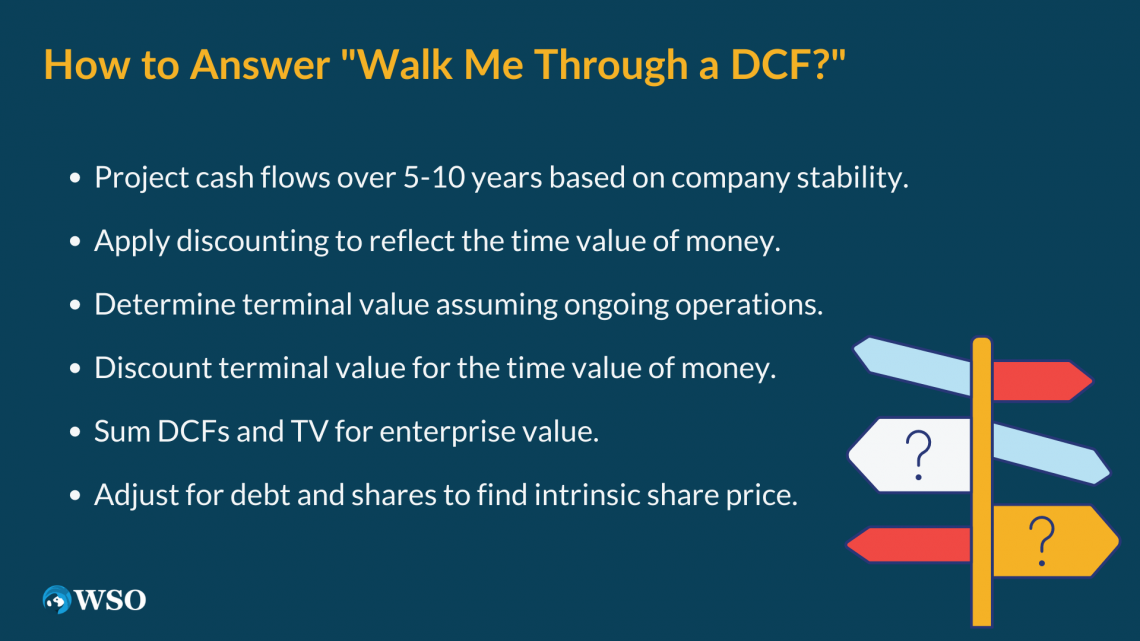In an interview, it is important to keep your technical overview at a high level. Start with a high-level overview and be ready to provide more detail upon request.

1. Project out cash flows for 5 - 10 years depending on the stability of the company
2. Discount these cash flows to account for the time value of money
3. Determine the terminal value of the company - assuming that the company does not stop operating after the projection window
4. Discount the terminal value to account for the time value of money
5. Sum the discounted values to find an enterprise value
6. Subtract Net Debt and divide by diluted shares outstanding to find an intrinsic share price

### A Detailed Walkthrough of the Answer

Now that you know how to tackle the question, we will take you through each step of the process in detail so you can be well-prepared in case your interviewers ask you to explain the steps.

The practical goal of a DCF is to ascertain whether the intrinsic value (based on present values) is higher or lower than the price the company is trading at on the market. A higher intrinsic value supports a buy decision, while a lower value supports a no-buy or sell.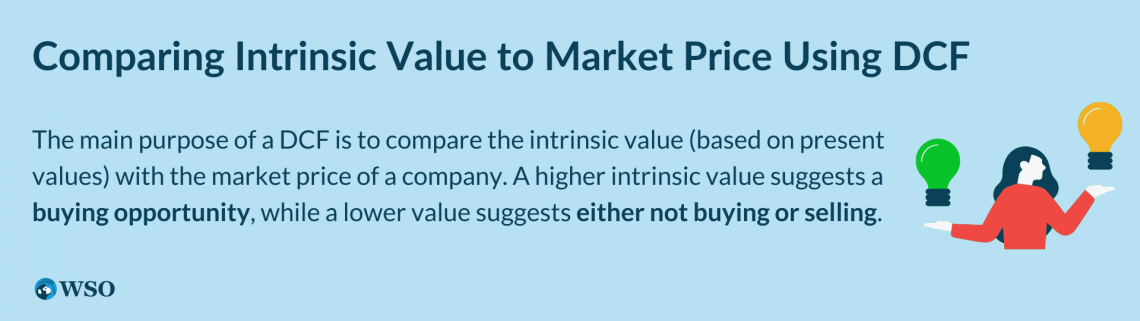Since DCFs are based on future cash flows, can a start-up with predominantly negative cash flow be a candidate for a DCF

This is a crucial point; most ideal candidates for DCFs are companies with a prolonged financial history - such as General Electric or Coca-Cola - as this makes future cash flows predictable.

Future projections act as a foundation for the entire DCF. Therefore, selecting Operating Data and Balance Sheets from the company will be beneficial for inputting forecasted FCF, debt, earnings, and equity values.

In some cases, you will not have access to projected values in company reports; for example, debt is something that you may not be able to forecast purely based on the projections in reports.

So understanding the appropriate proxies for specific variables in a DCF is key to getting an accurate output.

DCFs comprise four main stages, projecting unlevered FCF for five years, Calculating Terminal Value, Calculating the discount rate (Working Average Cost of Capital), and then calculating Equity value per share. WACC is the discount rate because FCF is unlevered.

It is essential to discount FCFs to their PVs with WACC as it accounts for the whole capital structure of the company.

### Step 1: Building a long-term Free Cash Flow Buildup

So how does this apply in an actual model? The most significant element in a DCF is its projected annual forecast section, where you can see the company’s cash flow buildup and calculate the net unlevered cash flows for each year.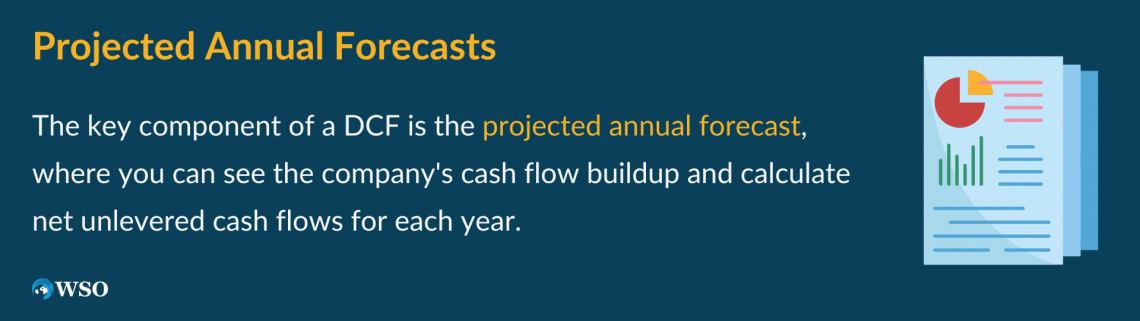Usually, even if the company is mature, we stick to 5 years to see cash flow buildup. To build the Free Cash Flow Buildup schedule, we must select Operating and Balance sheet data from the company reports.

The 5-year duration is a comfortable range for most investors as it is based on the logic that a company’s financials will not change dramatically in 5 years as much as they could in over ten years. Predictions over ten years are unlikely to be true and should be taken with a grain of salt.

The skeleton for Free Cash Flow Buildup for a forecasted period of 5 years should look something like this.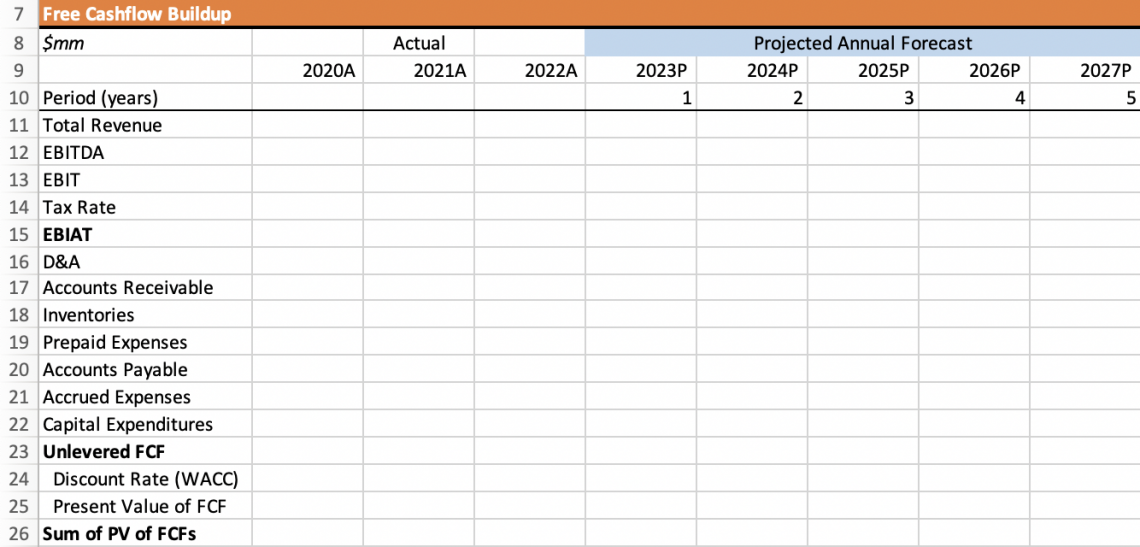The cash flow type used in DCF models is Unlevered cash flows, meaning the free cash flow is available to pay stakeholders in the company, such as equity holders and debt holders. This represents the industry norm and allows for an apples-to-apples comparison.

Free Cash Flow Formula explained:

Unlevered FCF = EBIT (1 – t) + DEPRECIATION & AMORTIZATION – CAPEX – NET CHANGE IN WC

EBIT means Earnings before Interest and Taxes (Net Income), which can be calculated by:

EBIT = Revenue - Cost of Goods Sold - Operating expenses

In Excel, since referencing “Accounts Receivable” should be negative, be sure to give values the correct sign within the formula when calculating Unlevered FCF. Incorrect signs may cause a mistake in your calculation, distorting the final DCF value.Other variables that should be negative are “inventories,” “paid expenses,” and “CAPEX.” When referring to the select balance sheet, if you input a formula and select the previous year to subtract from the consecutive year, Excel will automatically embed the sign.

The negative sign in Excel is displayed with brackets around the value: (x).

To calculate Unlevered FCF, input the selected balance sheet and data values into the model and add EBIAT, D&A, Working Capital Expenses, and CAPEX values.

In other words, referring to the worksheet, we utilize the formula = sum(15:22). Then, drag this value across the four consecutive years to calculate FCF for each year.

The WACC portion will be calculated later, so leave that blank for now. For PV of FCFs, we calculate this by dividing Unlevered FCF by (1+WACC for that year), which should produce the same value as the Unlevered FCF since we haven’t given a WACC value to the cell yet.

Next, drag this value across and ensure that the formula is still applied to each cell. Then, under the Sum of PV of FCFs, use the sum of the PV of FCF row to calculate this. In other words, concerning the worksheet, the formula should be =sum(E25:I25).

Select “Freeze Panes” on the cell to the right of Total Revenue to fill in the model while adjusting the zoom. Overall, if we filled out the worksheet, the model should look like this.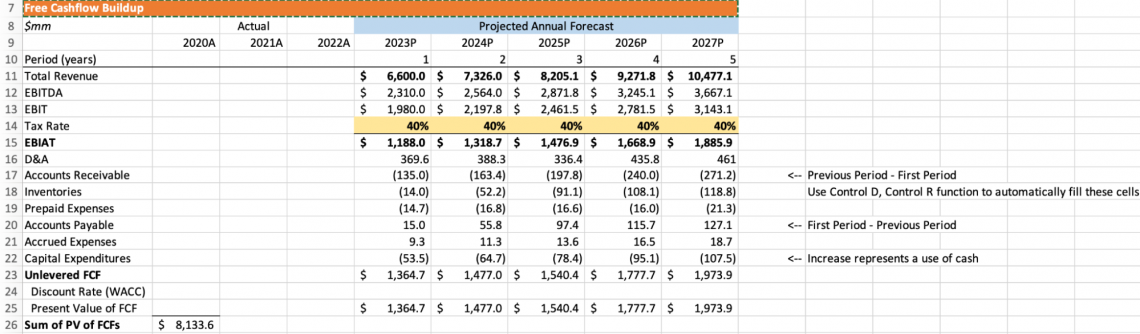This concludes Step 1 of the Analysis.

### Step 2: Calculate the Terminal Value

Before we build the model for our second step, unfreeze the panes to scroll freely and create a separate title beneath the Free Cash Flow Buildup called “Terminal Value.”

Terminal value is the value of future cash flows after the projection period. There are two methods for calculating this, educators favor one, and bankers favor the other. The first method is the “Exit Multiple,” and the second is “Perpetuity Growth.”

1. Exit multiple

This method assumes that the business will be sold. You find the exit multiple by finding the industry average multiple and multiplying it by the final year of revenue or final year EBITDA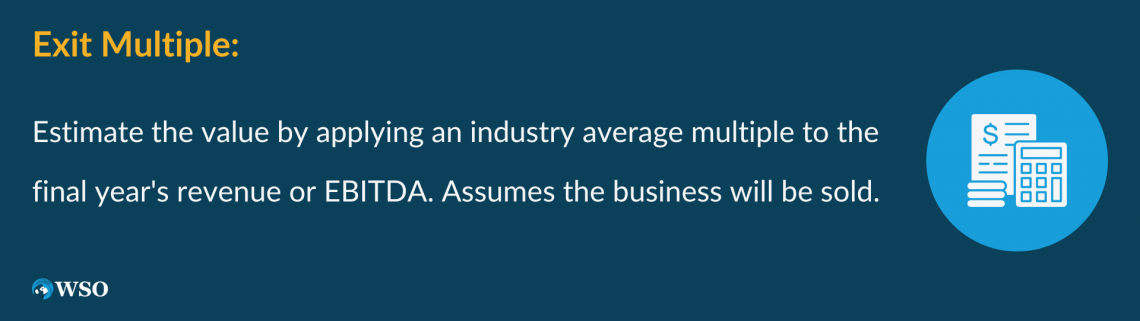Keep in mind that the final year of revenue is exclusively used if you are applying EV/Revenue, while EBITDA is used when EV/EBITDA is applied.

Conducting an excellent comparable analysis to choose a good industry multiple is essential to finding reasonable multiples. The best practice is to calculate the EBITDA/EBIT multiples implied by a perpetuity growth terminal value to see whether or not the value is reasonable.

2. Perpetuity Growth

The Perpetuity Growth method assumes that the final projected year of a company’s growth rate of free cash flows continues to grow indefinitely.

The applied rate is usually the GDP growth rate; most of the time, this rate is 2-3%, the average rate at which the US economy grows.

This rate makes sense as companies do not experience high growth rates for eternity. If they did, they would eventually outgrow the US economy, which would not be unrealistic and impossible.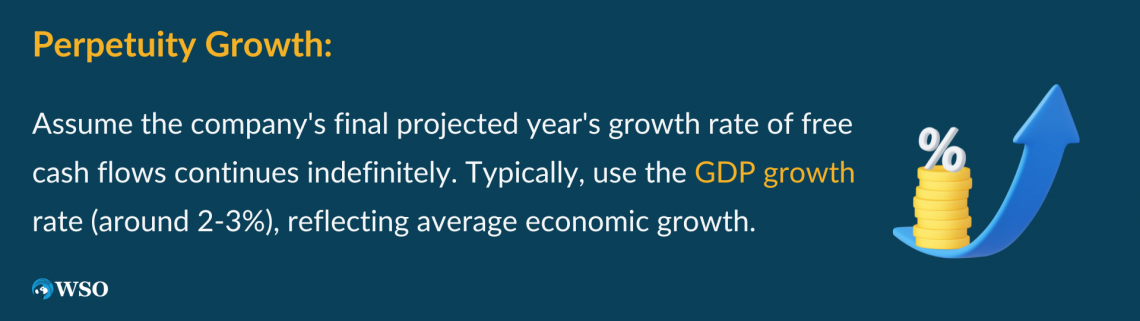As mentioned, “TV” is the value of future cash flows after the projection period and is calculated like this: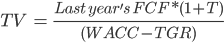R: discount rate
FCF: terminal free cash flow
G: growth rate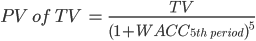In our example, Terminal Value is believed to exist in year 6, and indefinite growth refers to growth from year 6 onwards.

For the sake of this example, we will use the Perpetuity Growth method, as it is what most investment bankers use. First, we will assign a Long-Term Growth Rate of 4%. Then, using the FCF in year 5 and the Growth Rate (t), we can fill in our Terminal Value schedule by using the formula.As you can see, the TV and PV of the TV are both producing errors. This is because we haven’t assigned a WACC value to the TV or FCF Buildup Schedules. This concludes the second step and brings us into the next step, where we solve for WACC.

### Step 3: Discount the FCF and terminal value back to the present value using WACC.

WACC stands for Weighted Average Cost of Capital. It is the combined rate of return for all the capital holders of a company. The WACC is the rate used to discount TV back to PV; it is calculated by:

WACC= % equity*cost of equity+% of debt*After tax Cost of Debt )

A consensus in the industry is that the average WACC rate is 10%, less risky companies are rated around 6-9%, and riskier companies are 11-15%.Again, to begin this step, ensure the formatting and Freeze Panes are prepared before inputting any values. WACC also requires the “Total Debt” and “Total Equity” categories, which means you must research your company adequately to get your values.

Remember, our FCF buildup is based on unlevered cash flows, meaning the FCFs calculated are amounts available to all capital providers, not just the equity investors. Therefore, if we used the levered discount approach, the appropriate discount rate would be the cost of equity.

Therefore, we use WACC as our discount rate because we calculate the company’s cash flows before interest payments (EBIAT is before interest expense). WACC also allows for an apples-to-apples analysis. So we use it to bring our projections from 2022 onwards back to the present.

Now create a new title for our third Schedule titled “WACC” under the TV Schedule. The first thing we have to do is reference the share price; the price should reflect the value corresponding to the valuation date.

So if I create a valuation on 29/08/2022 for the Tesla.inc stock, the share price would be US\$285.12.

In terms of Diluted shares outstanding, what do we mean by that? Why can we not just use the basic share count on the front page of the company report? We have to use Diluted Shares as the company share count typically does not reflect the total claims of ownership of the company.

The main elements in this schedule are “Cost of Debt,” “Tax rate,” “After-Tax Cost of Debt,” and “Cost of Equity.” Why do we use the After-Tax Cost of Debt? Because we know that because we are using an unlevered cash flow analysis, our interest expense creates a real tax shield.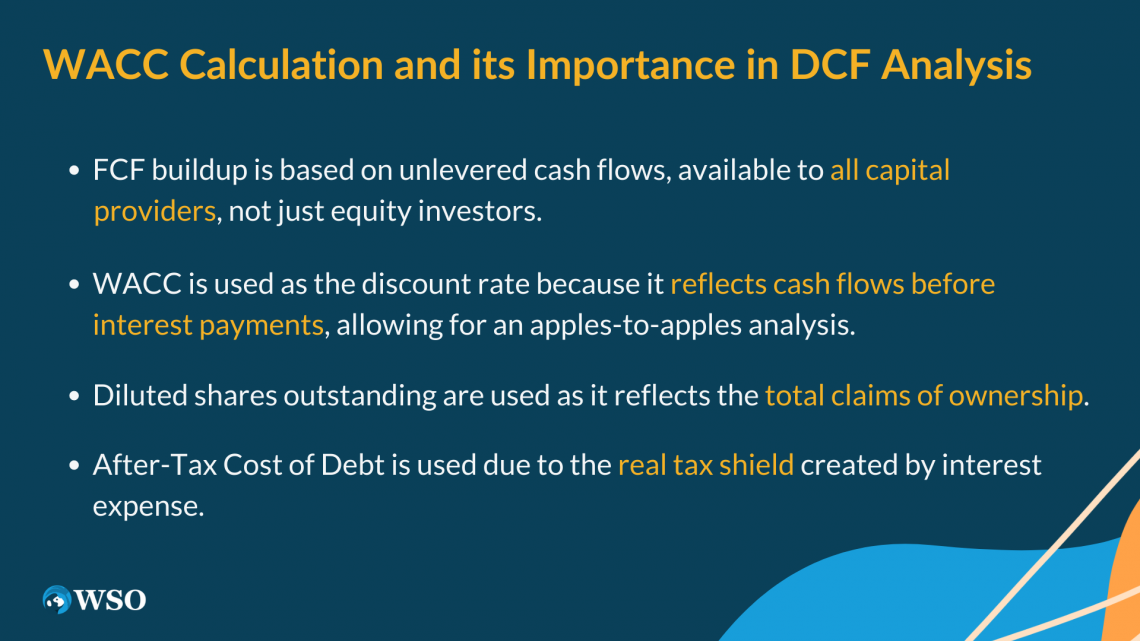The After Tax cost of debt represents the interest tax shield the company experiences by using debt in its capital structure. This is how the WACC calculation accounts for interest and tax expenses.

A rule to remember is if you are building a valuation for a company, “Cost of Debt” uses yield to worst. When dealing with comparable company debt, you use yield to maturity, agreed upon by most professionals.

“Cost of Equity,” on the other hand, is a highly debatable topic. This is because a combination of potential dividend payments and price appreciation make up this value. Practitioners commonly use the Capital Asset Pricing Model, so for this example, we will use that model.

The Capital Asset Pricing Model is calculated by the Risk-Free Rate plus Beta multiplied by the market risk premium.

Lastly, if you do not have forecasted figures for debt, book values for the current year act as a good proxy for future debt.

Share Price times diluted share count calculates total Equity. Total capital is the sum of these two variables. Find weighting by dividing these values by total capital.

The weighting should add up to 100%, and the result should look like this.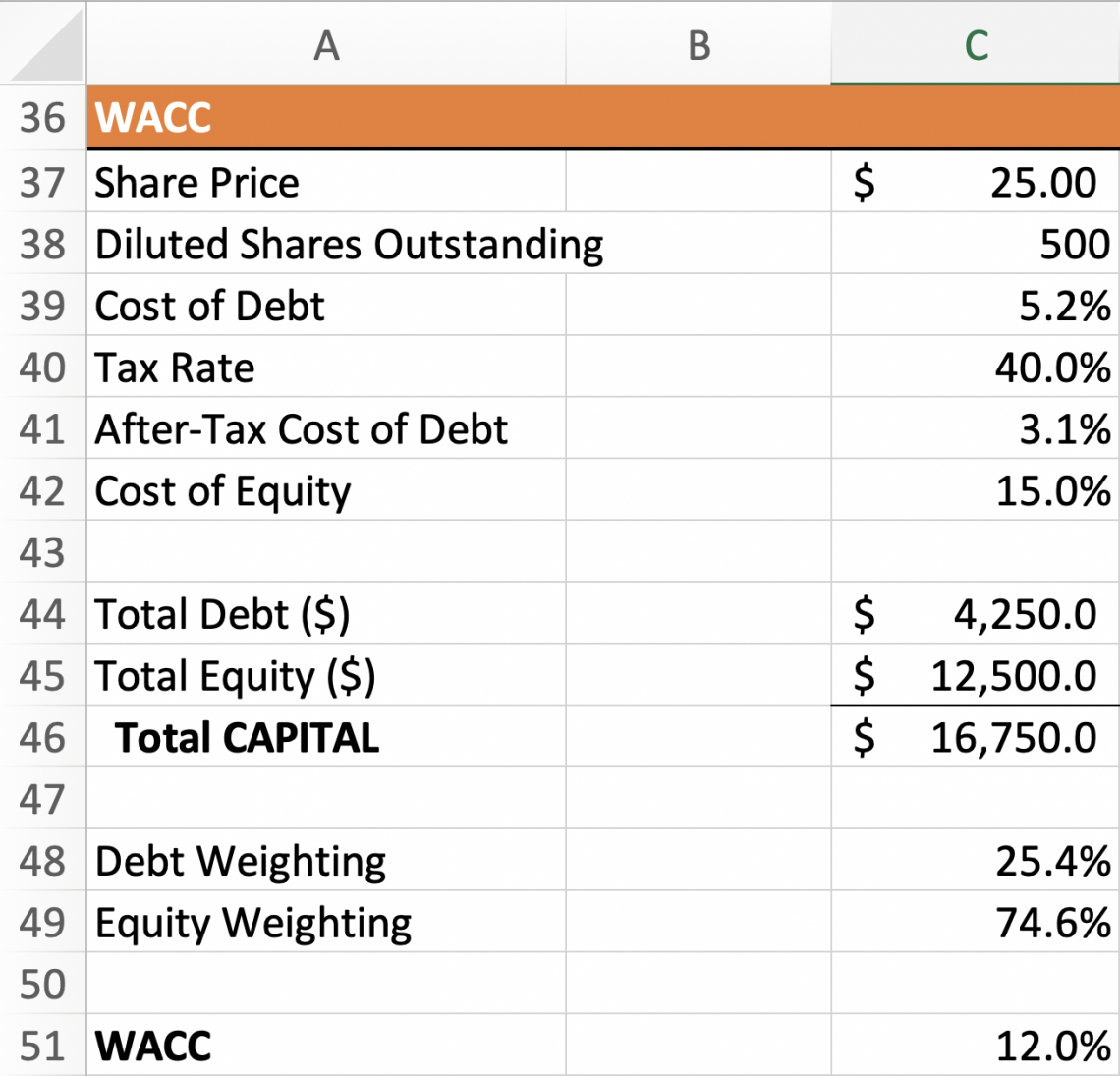By referring to the “WACC” formula, we can calculate WACC and plug the value into our previous schedules. The Excel formula to use to calculate WACC should be calculated: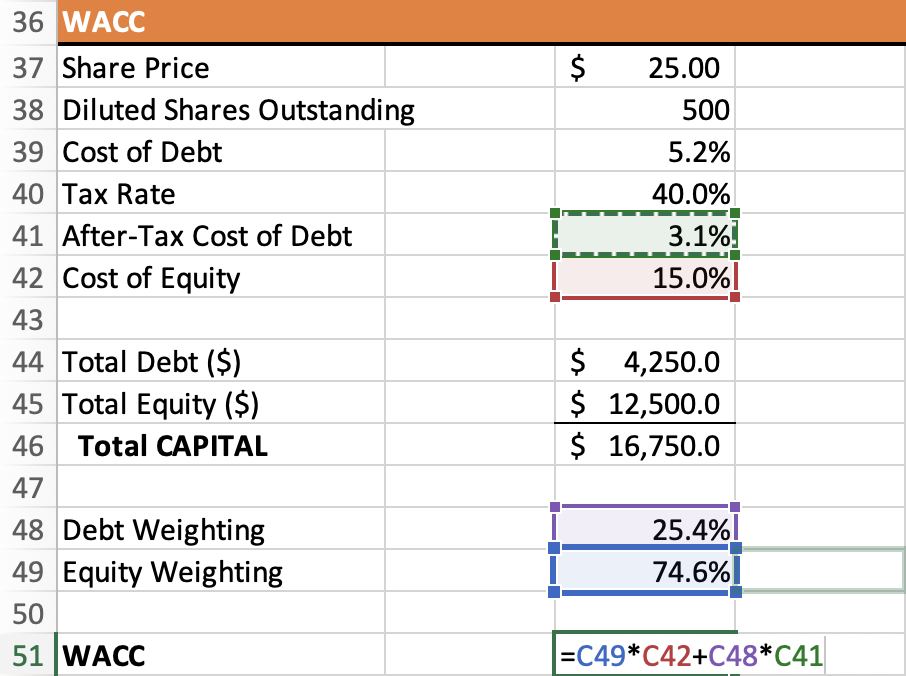With that, WACC should equal 12.0%. If we input this value into the previous schedules, the Present Values will begin to update because the cash flows now have a discount rate. So the new values for PV should look like this.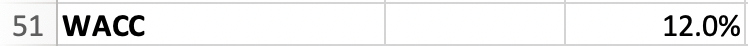1. FCF Buildup Schedule (Step 1 updated)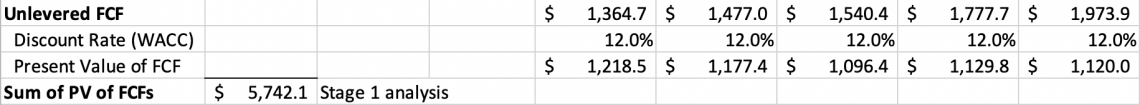Sum of PV of FCFs = \$5,742.1

2. TV Schedule (Step 2 updated)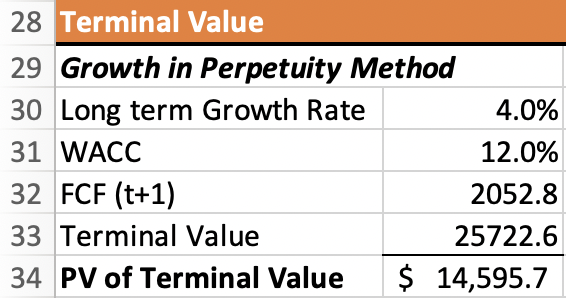PV of Terminal Value = \$14,595.7

For the “Terminal Value” cell, ensure the Excel formula has brackets for the denominator, as you can end up with very different values without them.

This concludes the end of step 3. To recap what we have just done, we have calculated the WACC value, which has acted as a discount rate in our previous two schedules, discounting our cash flows to their present values.

### Step 4: Calculating the Enterprise Value to Equity Value

For this step, format a new title called “Enterprise Value to Equity Value” under the WACC schedule.

The relevant elements in this schedule are

• “Enterprise value”
• “Net Debt”
• “Equity Value”
• “Diluted Shares Outstanding”
• “Equity Value per share”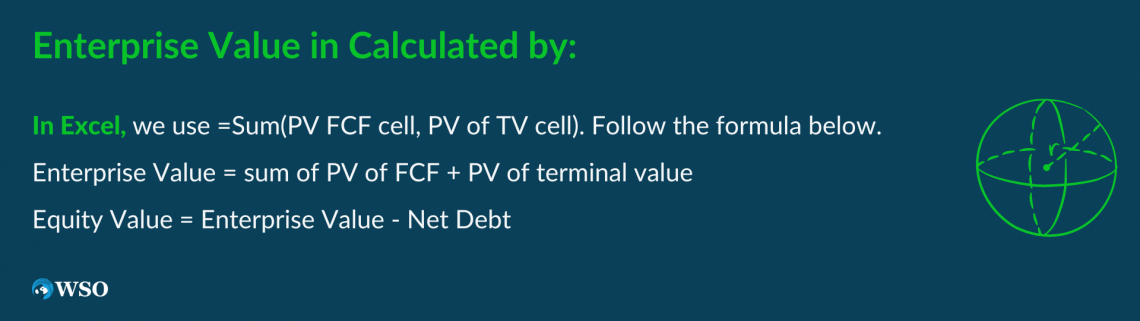Now that we have calculated the WACC value, we can calculate the “Enterprise Value”. To calculate Enterprise value, we sum up the present value of the free cash flows and terminal value to derive the enterprise value.

In Excel, we use =Sum(PV FCF cell, PV of TV cell). Follow the formula below.

Enterprise Value = sum of PV of FCF + PV of terminal value

After which, we subtract the net debt to calculate the equity value. In other words

Equity Value = Enterprise Value - Net Debt

But how do we calculate net debt? Well, here, we use the total gross amount of debt. This can include debt, minority interests, preferred stock, and anything that is a non-equity claim. In simple examples, with only “cash” and “debt,” remember to subtract cash from debt before using it.

The rationale here is that if we have excess liquidity, we subtract that from our outstanding debt to derive a lower debt value. Then, once we have Net Debt, we subtract it from Enterprise Value to get Equity value.

Next, we divide the equity value by outstanding shares to find the intrinsic value of each share, and we compare this value with the market price. If the market price is higher, the company shares are expensive; if the value is lower, the company sells at a discount.

Our Enterprise to Equity Value schedule should now look like this.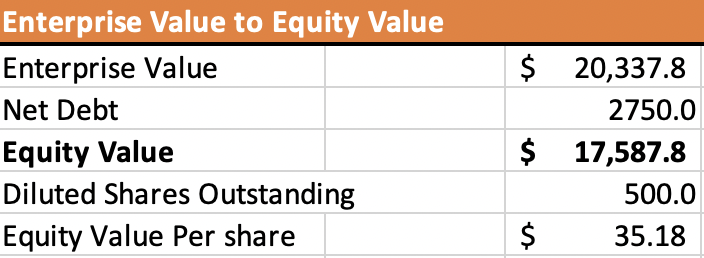Equity Value Per Share: \$35.18

Question: If the company’s stock is trading at \$30, would you buy or sell this company? Why?

Answer: You would buy this company because it has a higher intrinsic value than what it is worth on the market, so if I bought the stock, I would benefit from buying it at a discounted price.

Now that we know how a DCF works start-to-finish, we can answer the interview question “walk me through a DCF” through these steps:

1. Forecast Free Cash Flows for five years.

2. Determine the company’s terminal value; this assumes that the company continues operating after the projection window(beyond year 6).

3. Calculate our discount rate (WACC).

4. Discount our FCFs back to their PVs using the discount rate to account for the time value of money.

5. Find Enterprise Value through the PVs of FCF and PV of TV.

6. Subtract Net Debt and divide by diluted shares outstanding to find Equity Value per Share price to compare to the Market Share price to make a buy/sell decision.

### FAQ: Follow-up Questions Interviewers may ask### The Only Program You Need to Land in High Finance Careers

The most comprehensive curriculum and support network to break into high finance.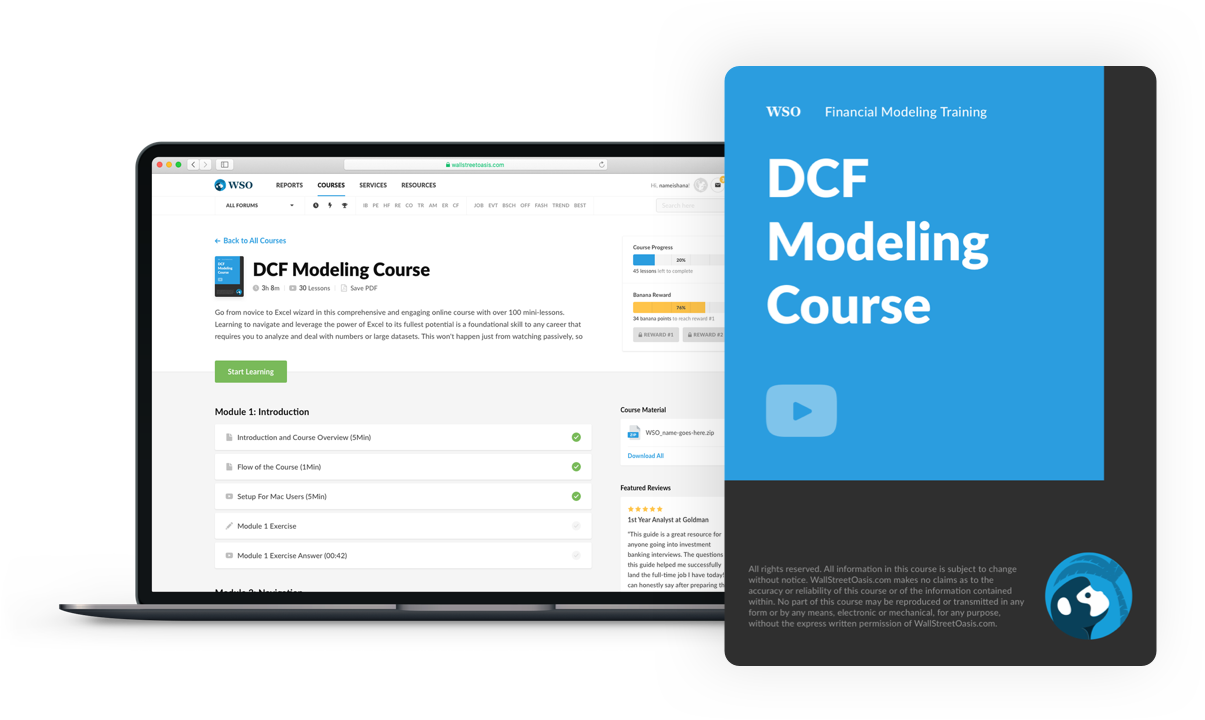### Everything You Need To Master DCF Modeling

To Help You Thrive in the Most Prestigious Jobs on Wall Street.

Researched & Authored by Sevriano Battista | LinkedIn

Reviewed and Edited by Kevin Wang | LinkedIn